# How Do You Convert Minutes to Hours in Google Sheets? From Minutes to Hours

When you are working with a large dataset, the conversion of minutes to hours might become a primary need. This might not be required in all types of datasets, but suppose you are handling an employee dataset and want to know which employee clocked for how many hours in minutes, then this conversion plays a major role. And to help spreadsheet users with this, Google Sheets allows users to convert minutes to hours using various methods. Let us discuss all the methods with the help of which we can convert minutes to hours using Google Sheets Tips. Scroll down to find out more.

## How Do You Change Minutes to Hours?

There are various methods with the help of which we can easily convert minutes to hours. But one of the easiest methods with the help of which we can convert the minutes to hours is to divide the minutes by 60. The detailed steps on how to use this formula in the spreadsheet are outlined below:

• 2nd Step: Now on the homepage, move to the cell where you need to convert the minutes to hours.
• 3rd Step: Here, type the formula “=cellrange/60“. In this example, the formula is a2/60.
• 4th Step: Press the “Return” key and you will find the results as shown in the image given below.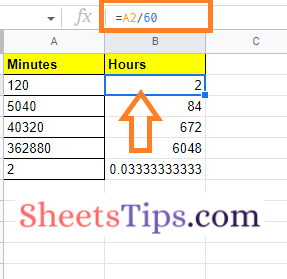Now drag the formula applied cell to other parts of the cell and you will find the minutes being converted to the hours in the spreadsheet.

When you don’t want to use any functions and convert the given data instantly, you can opt for this method.

However, as discussed above, there are various other methods that can be used to convert minutes to hours in Google Sheets. Let us discuss those methods in the below section.

### How to Convert Minutes to Hours in Google Sheets Using the Convert Function?

Using the CONVERT function, one can easily convert the given minutes into hours. The steps to use the CONVERT function in the spreadsheet are given below:

• 2nd Step: Now on the homepage, move to the cell where you want to display the results of the minutes to hours conversion.
• 3rd Step: Enter the formula =CONVERT (A2, “min”, “hr”) here.
• 4th Step: Press the “Return” key and you will find the results shown below.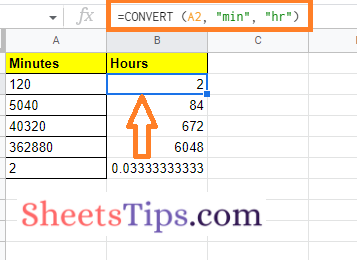Formula Explanation The syntax of = CONVERT (value, start_unit, end_unit) The value here represents the cell range, and start_unit is the unit for which the unit needs to be converted. The end-unit is the unit that shows the result of the conversion. In our case, the start-unit is minutes and the end_unit is minutes.

### How to Use the Text Function to Convert Minutes to Hours?

Follow the steps as outlined below to convert the given minutes to hours using the TEXT function on Google Sheets:

• 2nd Step: Now on the homepage, move to the cell where you want to apply the formula.
• 3rd Step: Type the formula as “=TEXT(A2/60/24,“hh:mm”)“. Here, A2 refers to the cell range.
• 4th Step: Press the “Enter” key and you will see the results as shown in the image given below.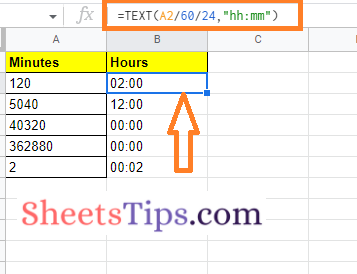### How to Convert Minutes to Hours using the Time Function?

The steps to convert minutes to hours using the TIME function are given below:

• 2nd Step: Now on the homepage, click on the cell where you want to enter the min to hr formula.
• 3rd Step: Enter the formula as =TIME (0,A2,0). Here, A2 refers to the cell range. Based on your cell range, you can optimize this formula.
• 4th Step: Press the “Enter” key and you will find the results as shown in the image given below.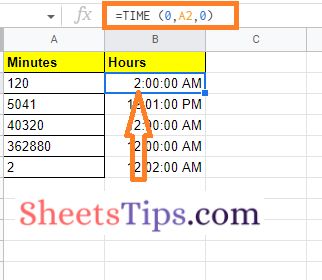### How to Convert Minutes to Hours using a Custom Date and Format?

The steps to convert minutes to hours using the custom date and format in Google Sheets are given below:

• 2nd Step: Now on the homepage, click on the cell where you want to enter the conversion formula.
• 3rd Step: Here, enter the formula “=A2/60/24“.
• 4th Step: Press the “Enter” key and you will find the results as shown in the image given below.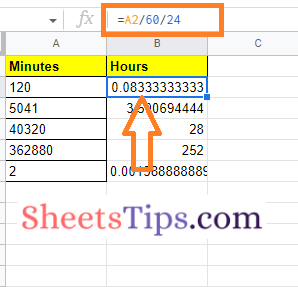• 5th Step:  Select column B.
• 6th Step: Click on the “Format” tab and choose “Custom date and time“.
• 7th Step: Choose “Hour:Minute” format from the drop down.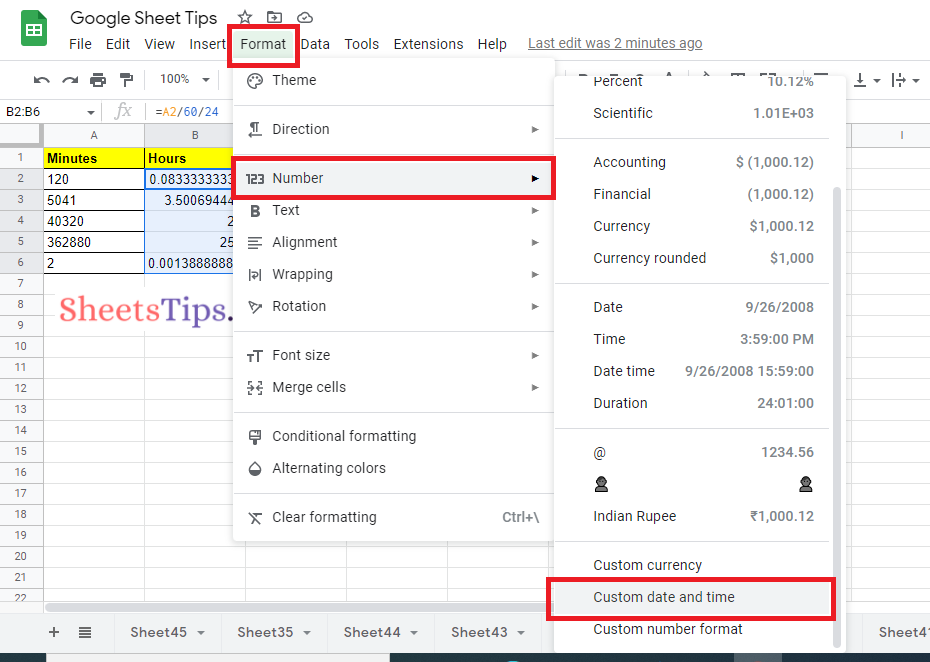• 8th Step: Press the “Done” button and you will find the results as shown below.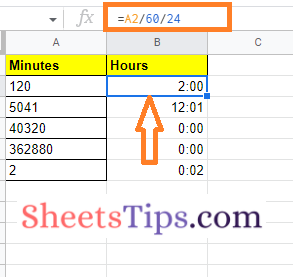Though the need of converting minutes to hours is not required every day, we might fall under the situation for conversions to make the data more presentable. So depending on the urgency, one can use any of the methods presented above to convert their data on the Google Sheets.